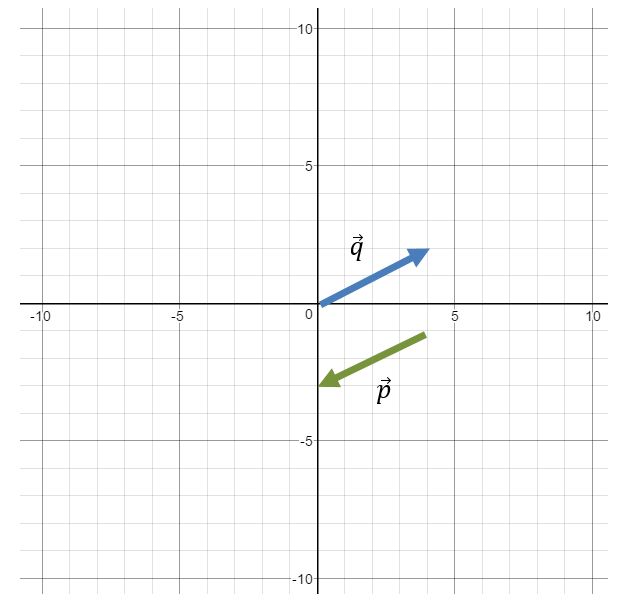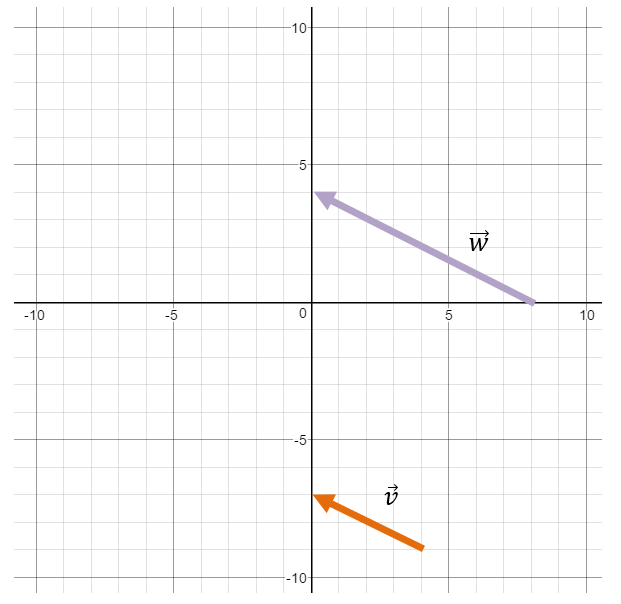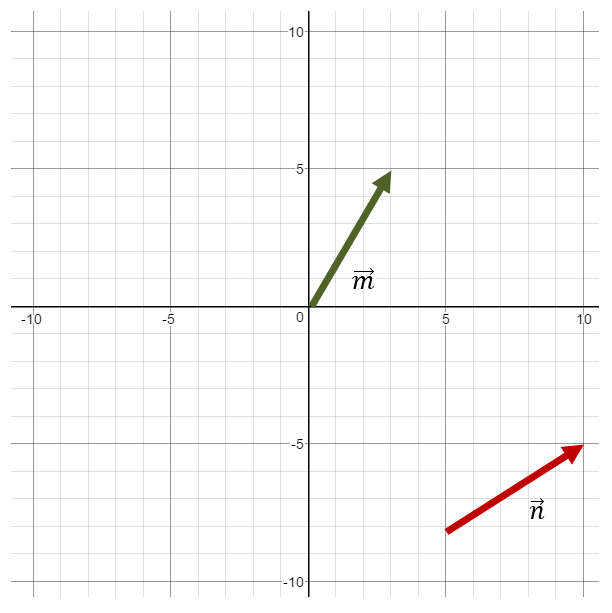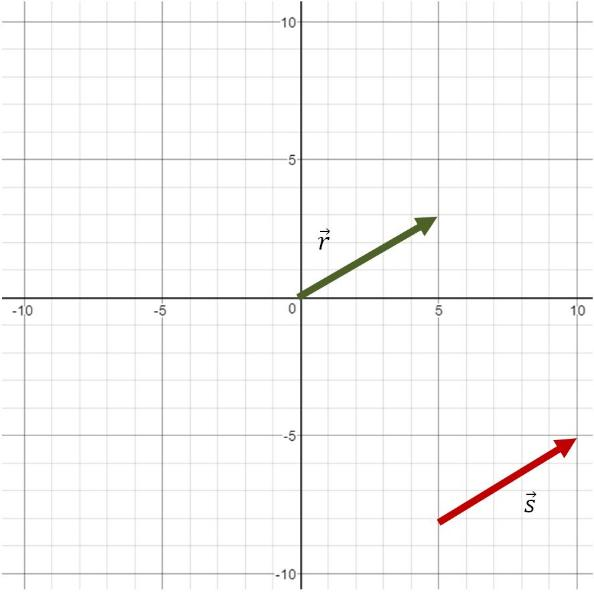# Equivalent vectors

### Equivalent vectors

There are times when we are interested to see whether multiple vectors are considered equivalent or not. When we say two or more vectors are equivalent vectors, we simply mean that the vectors that are being compared have both of the following equivalent -- the direction and the magnitude. We will work on some relevant examples in this section.

#### Lessons

• 1.
Are $\vec{p}$ and $\vec{q}$ equivalent vectors?• 2.
Are $\vec{w}$ and $\vec{v}$ equivalent vectors?• 3.
Are $\vec{m}$ and $\vec{n}$ equivalent vectors?• 4.
Are $\vec{r}$ and $\vec{s}$ equivalent vectors?